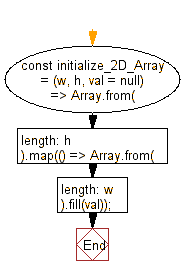# JavaScript: Initialize a two dimension array of given width and height and value

## JavaScript fundamental (ES6 Syntax): Exercise-53 with Solution

Write a JavaScript program to Initialize a two dimension array of given width and height and value.

• Use Array.from() and Array.prototype.map() to generate h rows where each is a new array of size w.
• Use Array.prototype.fill() to initialize all items with value val.
• Omit the last argument, val, to use a default value of null.

Sample Solution:

JavaScript Code:

``````//#Source https://bit.ly/2neWfJ2
const initialize_2D_Array = (w, h, val = null) =>
Array.from({ length: h }).map(() => Array.from({ length: w }).fill(val));

console.log(initialize_2D_Array(2, 2, 0));
console.log(initialize_2D_Array(3, 3, 3));
```
```

Sample Output:

```[[0,0],[0,0]]
[[3,3,3],[3,3,3],[3,3,3]]
```

Pictorial Presentation:Flowchart:Live Demo:

See the Pen javascript-basic-exercise-1-53 by w3resource (@w3resource) on CodePen.

Improve this sample solution and post your code through Disqus

What is the difficulty level of this exercise?

Test your Programming skills with w3resource's quiz.

﻿

## JavaScript: Tips of the Day

Chunks an array into n smaller arrays

Example:

```const tips_chunkIntoN = (arr, n) => {
const size = Math.ceil(arr.length / n);
return Array.from({ length: n }, (v, i) =>
arr.slice(i * size, i * size + size)
);
}
console.log(tips_chunkIntoN([1, 2, 3, 4, 5, 6, 7,8], 4));
```

Output:

```[[1,2],[3,4],[5,6],[7,8]]
```